The Sobel transform is an edge detection algorithm. Edges are identified by calculating the difference in surrounding pixels. Pixels with large differences are likely to be edges. This is why the edges are white and non-edges are black. In 8-bit grayscale, white is 255 and black is 0.

Fig. 1 - Result of running OpenCV sobel demo on Trees.jpg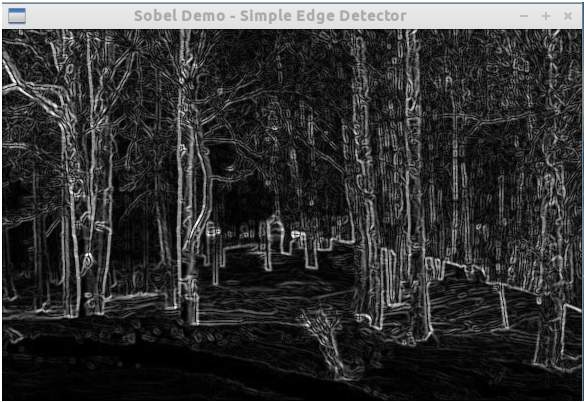To better understand the Sobel transform, linear filtering, and kernels/masks, I implemented the Sobel transform manually. The results were not as a good as the OpenCV implementation, but it was reasonable.

Initially, I implemented simple 2x1 and 1x2 kernels to detect vertical and horizontal edges. After that I computed the gradient of the vertical and horizontal results. This approach to try 2x1 and 1x2 kernels was based on an example from the Computer Vision module of the Udacity: Introduction to AI course.

Fig. 2 Result of linear filter with a simple 2x1 kernel to detect vertical edges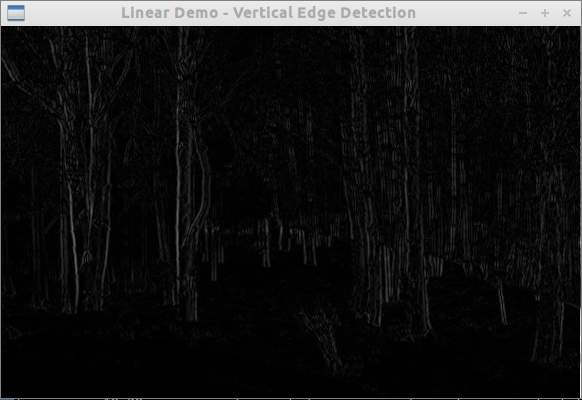Fig. 3 Result of linear filter with a simple 1x2 kernel to detect horizontal edges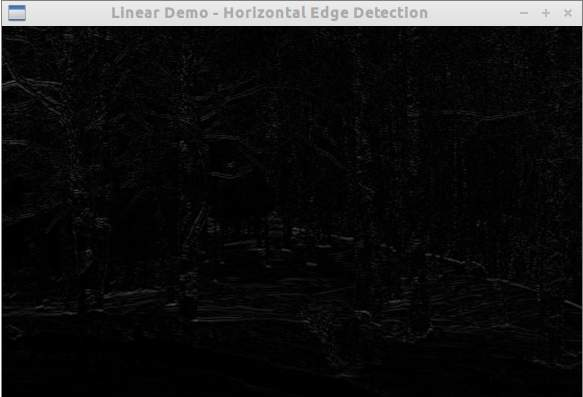Fig. 4 Result of computing gradient of both vertical and horizontal computations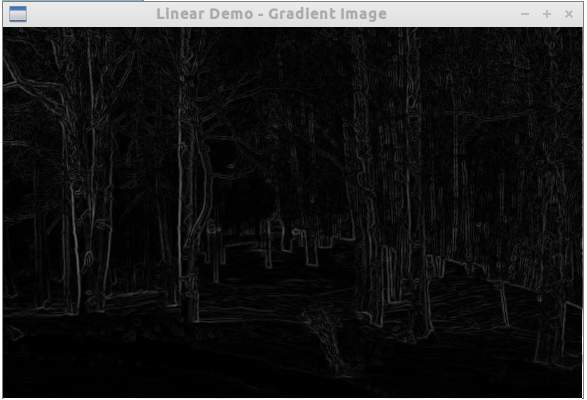It took some trial and error to even get the simple kernels working. Part of this was due to inexperience with OpenCV, so this turned out to be a good introduction. One big mistake was cloning the grayscale image for each gradient (grad_x and grad_y) instead of creating an empty matrix with appropriate value type. This lead to overflow errors since both matrices used 8-bit unsigned ints.

Fig. 5 Result of overflowing calculations when computing vertical edges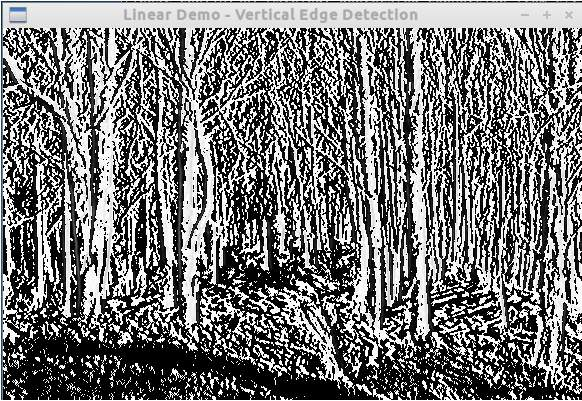Fig. 6 Result of accidentally miscalculating absolute value of differences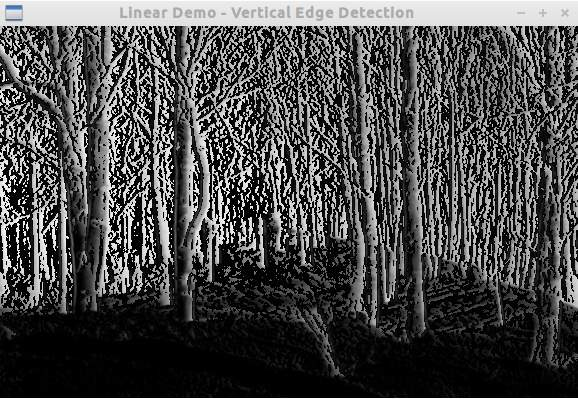After fixing the kinks of the linear filter using simple kernels, I implemented the sobel kernel. This required modifications and improvements from the simple kernel implementation. For instance, with the 2x1 and 1x2 kernels, values could be access and computed values using pointers (assuming that memory is always allocated contiguously in OpenCV). And for the horizontal edge computation the matrix can simply be transposed.

To compute the 3x3 kernels that the Sobel transform uses, the “at” method was to access the adjacent pixel values instead of pointer access. The Udacity course also discussed the Sobel kernel briefly, but glossed over how values were computed. The OpenCV tutorial on masking operations helped immensely in this regard. The key is realizing that the center pixel represented the current pixel.

Fig. 7 Results of my naive sobel transform implementation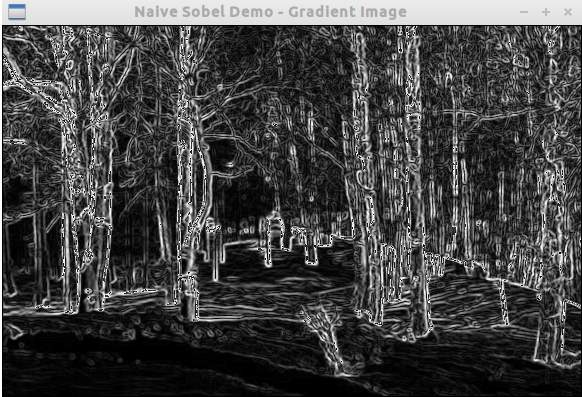I’ve also been working on javascript implementations of various Computer and Machine vision algorithms. The early demos can be viewed here.